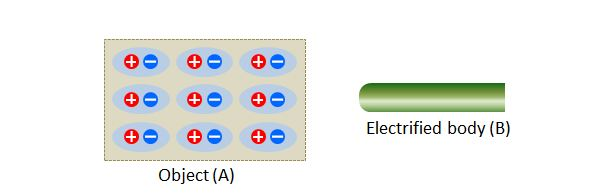# Electrostatic inductionIn the above diagram, $(A)$ is the distribution of electrical charges in an object, being influenced by a nearby electrified body $(B).$ What are the object $(A)$ and the type of charge on $(B),$ respectively?

×